Next: Black-body radiation Up: Quantum statistics Previous: Quantum statistics in the

## The Planck radiation law

Let us now consider the application of statistical thermodynamics to electromagnetic radiation. According to Maxwell's theory, an electromagnetic wave is a coupled self-sustaining oscillation of electric and magnetic fields which propagates though a vacuum at the speed of light,. The electric component of the wave can be written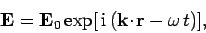(631)

whereis a constant,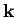is the wave-vector which determines the wavelength and direction of propagation of the wave, andis the frequency. The dispersion relation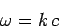(632)

ensures that the wave propagates at the speed of light. Note that this dispersion relation is very similar to that of sound waves in solids [see Eq. (508)]. Electromagnetic waves always propagate in the direction perpendicular to the coupled electric and magnetic fields (i.e., electromagnetic waves are transverse waves). This means that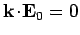. Thus, onceis specified, there are only two possible independent directions for the electric field. These correspond to the two independent polarizations of electromagnetic waves.

Consider an enclosure whose walls are maintained at fixed temperature. What is the nature of the steady-state electromagnetic radiation inside the enclosure? Suppose that the enclosure is a parallelepiped with sides of lengths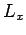,, and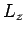. Alternatively, suppose that the radiation field inside the enclosure is periodic in the-,-, and-directions, with periodicity lengths,, and, respectively. As long as the smallest of these lengths,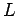, say, is much greater than the longest wavelength of interest in the problem,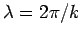, then these assumptions should not significantly affect the nature of the radiation inside the enclosure. We find, just as in our earlier discussion of sound waves (see Sect. 7.12), that the periodicity constraints ensure that there are only a discrete set of allowed wave-vectors (i.e., a discrete set of allowed modes of oscillation of the electromagnetic field inside the enclosure). Let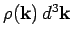be the number of allowed modes per unit volume with wave-vectors in the rangeto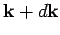. We know, by analogy with Eq. (514), that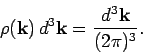(633)

The number of modes per unit volume for which the magnitude of the wave-vector lies in the rangetois just the density of modes,, multiplied by the volume'' in-space of the spherical shell lying between radiiand. Thus,(634)

Finally, the number of modes per unit volume whose frequencies lie betweenandis, by Eq. (632),(635)

Here, the additional factor 2 is to take account of the two independent polarizations of the electromagnetic field for a given wave-vector.

Let us consider the situation classically. By analogy with sound waves, we can treat each allowable mode of oscillation of the electromagnetic field as an independent harmonic oscillator. According to the equipartition theorem (see Sect. 7.8), each mode possesses a mean energy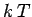in thermal equilibrium at temperature. In fact,resides with the oscillating electric field, and anotherwith the oscillating magnetic field. Thus, the classical energy density of electromagnetic radiation (i.e., the energy per unit volume associated with modes whose frequencies lie in the rangeto) is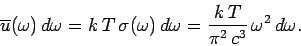(636)

This result is known as the Rayleigh-Jeans radiation law, after Lord Rayleigh and James Jeans who first proposed it in the late nineteenth century.

According to Debye theory (see Sect. 7.12), the energy density of sound waves in a solid is analogous to the Rayleigh-Jeans law, with one very important difference. In Debye theory there is a cut-off frequency (the Debye frequency) above which no modes exist. This cut-off comes about because of the discrete nature of solids (i.e., because solids are made up of atoms instead of being continuous). It is, of course, impossible to have sound waves whose wavelengths are much less than the inter-atomic spacing. On the other hand, electromagnetic waves propagate through a vacuum, which possesses no discrete structure. It follows that there is no cut-off frequency for electromagnetic waves, and so the Rayleigh-Jeans law holds for all frequencies. This immediately poses a severe problem. The total classical energy density of electromagnetic radiation is given by(637)

This is an integral which obviously does not converge. Thus, according to classical physics, the total energy density of electromagnetic radiation inside an enclosed cavity is infinite! This is clearly an absurd result, and was recognized as such in the latter half of the nineteenth century. In fact, this prediction is known as the ultra-violet catastrophe, because the Rayleigh-Jeans law usually starts to diverge badly from experimental observations (by over-estimating the amount of radiation) in the ultra-violet region of the spectrum.

So, how do we obtain a sensible answer? Well, as usual, quantum mechanics comes to our rescue. According to quantum mechanics, each allowable mode of oscillation of the electromagnetic field corresponds to a photon state with energy and momentum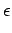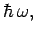(638)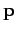(639)

respectively. Incidentally, it follows from Eq. (632) that(640)

which implies that photons are massless particles which move at the speed of light. According to the Planck distribution (602), the mean number of photons occupying a photon state of frequencyis(641)

Hence, the mean energy of such a state is given by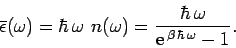(642)

Note that low frequency states (i.e.,) behave classically: i.e.,(643)

On the other hand, high frequency states (i.e.,) are completely frozen out'': i.e.,(644)

The reason for this is simply that it is very difficult for a thermal fluctuation to create a photon with an energy greatly in excess of, sinceis the characteristic energy associated with such fluctuations.

According to the above discussion, the true energy density of electromagnetic radiation inside an enclosed cavity is written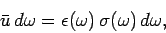(645)

giving(646)

This is famous result is known as the Planck radiation law. The Planck law approximates to the classical Rayleigh-Jeans law for, peaks at about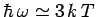, and falls off exponentially for. The exponential fall off at high frequencies ensures that the total energy density remains finite.Next: Black-body radiation Up: Quantum statistics Previous: Quantum statistics in the
Richard Fitzpatrick 2006-02-02